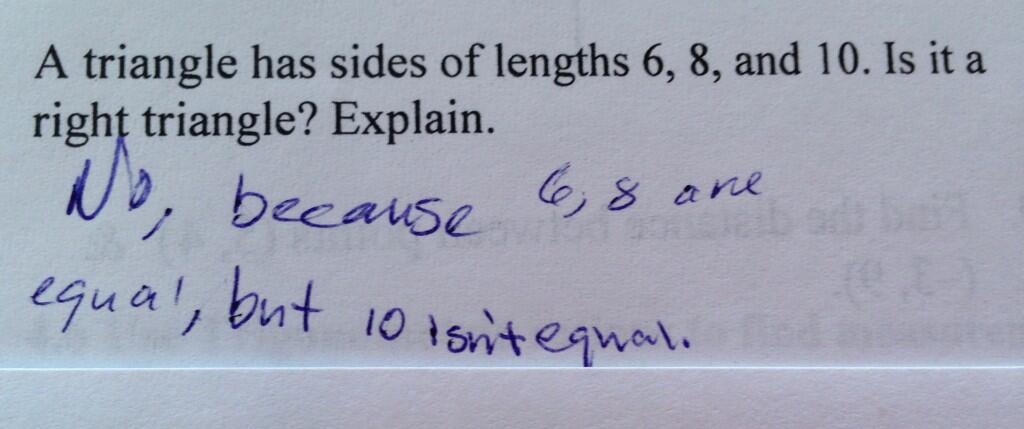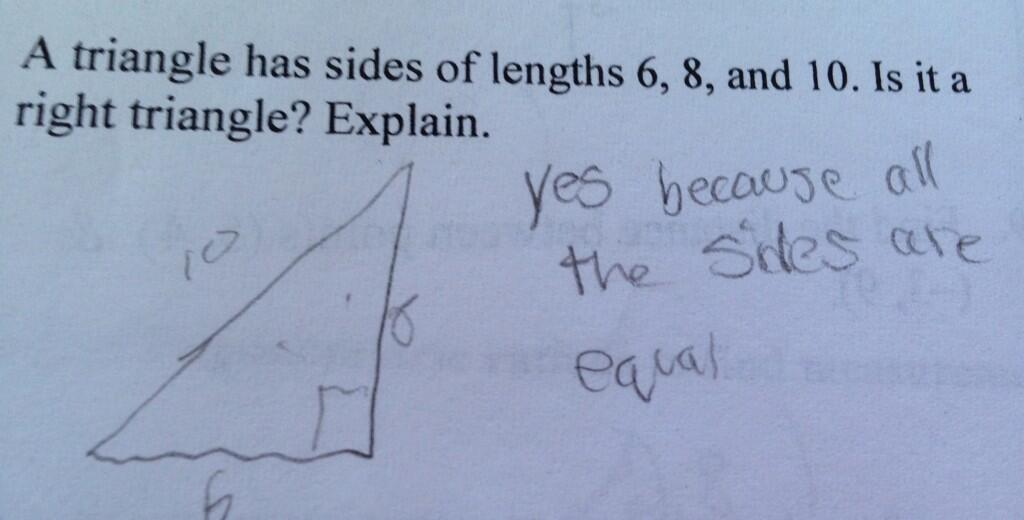Categories

# “All the sides in the 6, 8, 10 triangle are equal.”What interesting mistakes! Let’s make everything that’s puzzling about these explicit.

“6, 8 are equal, but 10 isn’t equal.”

• Does this mean that 6 and 8 are equal to each other? Or that 6 is equal, 8 is equal, but 10 is not equal. (To what???)
• What on Earth does it mean that 10 isn’t equal!
• What exactly does this student think “right triangle” means? Does he think it means that all the sides are equal?

“Yes, because all the sides are equal.”

• How? You drew a picture showing that the triangle has sides of length 6, 8 and 10!
• So a right triangle needs to have all equal sides?

This is mysterious to me, but what’s important is to not dismiss these students as hopelessly confused. Take the second mistake. What we’ve discovered is that you can know a lot and still think that a 6, 8, 10 triangle has all equal sides. That’s really cool!

As far as shedding light on these mistakes, I’m really having trouble coming up with anything that makes sense. I’d say that the top student is not saying that 6 and 8 are equal to each other, but then what is that student saying?

## 8 replies on ““All the sides in the 6, 8, 10 triangle are equal.””suevanhattumsays:

Seems to me we have to ask the students. I am fascinated.

What’s even more stunning is the conflation. Is it a right triangle? No, because they are EQUAL. They are confusing isosceles/equilateral in some way with right triangle.

Because 6^2+8^2=10^2 is that what they are thinking when they state the sides are equal?kmorrow-leongsays:

I feel that learners look for words to classify distinctions that they observe. In math, because of its level of precision, there often aren’t enough words. “Equal” is one of the few words that can represent “sameness. “. What is the same in a 6-8-10 triangle? I’d guess the observation is kind of an interesting view into the student’s thinking. For one student, they form a set of side lengths that is “the same” as other triangles they have seen. For bith students, particularly the other, like suevanhattum, I’d want to ask.

Maybe the second student’s understanding is that it is a right triangle because the sides are multiples of (equal to?) a 3, 4, 5 triangle, which the student has learned is a right triangle.

If I saw this in my classroom I might say – this student is not able to express his/herself in writing. Well, at least for the 2nd example.
The student understands that the Pythagorean Thm is important here and it involves an equality (equation) and the equation is true in this case. 6^2 + 8^2 = 10^2.

The first answer leads me to believe that the student doesn’t understand equality at all.

I wonder if Student 1 could be being sucked in to one of the common errors of proportional reasoning. Perhaps this kid recognizes a 3, 4, 5 triangle as right, but thinks that the “next” right triangle in the pattern would be a 6, 7, 8 triangle? So they like the 6 and 8 but not the 10? I’m stretching here, though… I like kmorrow-leong’s idea that “equal” is meaning “the same as” or “works” but am struggling to think what the 6 and 8 “work” in that the 10 doesn’t.Laura C.says:

That sounds like a possibility. The 1st student also seems to have that idea that = means “here comes the answer” or “this is the next thing in the series.”